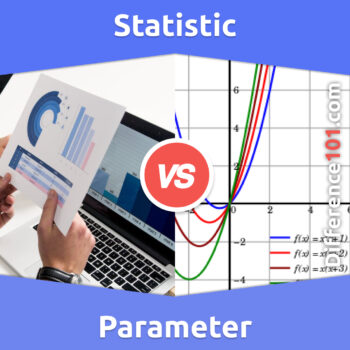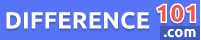# Parameter vs. Statistic: What’sThe Difference Between Parameter And Statistic?

What's the difference between a parameter and a statistic? We will explain the key differences between these two concepts, their pros and cons, and provide examples of when you would use each one. Read more here.Parameter vs. Statistic: In statistics vocabulary, we often hear of these terms, parameter and statistic, which both play an important role in determining the sample size. These two terms are often confused as people think they are the same. The main difference between parameter and statistic is that statistics is a summary of a small group known as the population, while the parameter is drawn from the measurement of the unit present within the population.

Let’s take a closer look at Statistic versus. Parameter

## What Is a Parameter?If we look at the parameter meaning, it is a numerical value drawn from the population’s elements. It can also be referred to as the fixed characteristics of the population. The numerical value of the parameter remains unchanged because to know the parameter, every number of the population is surveyed. A parameter is also known as the population parameter because it is the data obtained from the entire population. In statistics, a parameter may not be possible for every research, but for some, they are possible, for example, If there is a database for the number of registered votes in a state. Data collected from the database can be used to ask questions and calculate the parameters of the entire population of registered voters.

👉  Discrete Data vs. Continuous Data: What Is the Difference Between Discrete and Continuous Data?

## What Is Statistic?If we look at the statistic meaning, it can be defined as a numerical value obtained or drawn from the sample of the data. A statistic is a descriptive statistical measure and function of the sample observation. A sample can be defined as a fraction of the population that represents all the population’s characteristics. This sample is taken from the population to study, and the data produced by the perspective measured of that sample is the statistic. The sample has almost all the inferred population characteristics because it is impossible to study the entire population. Statistic is also famous for being a “sample statistic” because it is drawn from the sample. The sample statistic is used to collect information about every individual. However, the data may vary from sample to sample.

👉  Java vs. JavaScript: What’s The Difference Between JAVA and JavaScript?

## Parameter vs. Statistic Similarities

• Both of these terms are well-known in the statistic vocabulary
• Whether it is parameter or statistic, both are used to determine the sample size.
👉  Solute vs. Solvent: What’s The Difference Between Solute And Solvent?

## Parameter vs. Statistic Examples

### Parameter Examples

• Mean weight of all German Shepherd dogs
• The standard deviation of all transaction times in a particular bank
• The median income of a county

### Statistic Examples

• Mean weight of a random sample of 150 German Shepherds
• The standard deviation of a random sample of 700 transaction times at that bank
• The median income of a random sample of 60 from that county

## Parameter Pros and Cons### Pros of Parameter

• Parameters are a very important component of statistical analysis because these are the numerical values which can tell about the characteristics of the whole population.
• Parameter plays an important role in quantitative research because it allows the researchers to understand the behavior of the data in different conditions.

### Cons of Parameter

• Obtaining parameters from the larger population is very expensive and also consumes a lot of time.
• In some cases, like the national census, parameters are inaccurate as they cannot measure an entire population.

## Statistic Pros and Cons### Pros of Statistic

• Statistic helps in presenting bulk data in definite and precise form. As it can evaluate the claims based on quantitative evidence.
• Statistic makes forecasting and trends easy and also provides conclusion and comparison of the data.

### Cons of Statistic

• If the statistical methods are not done by the experts, there are high chances of errors in the data.
• The statistic cannot provide a fine comparison if the data is not uniform and homogenous.

## Comparison Chart## Conclusion

Parameter and statistics are the two terms used to determine the sample size. If we look at the parameter vs. statistic differences, these terms are used for different measurements and provide different results. Statistics is the numerical value of a small population group, while the parameter is the entire characteristics of the target population. The parameter is obtained from the unit measurement of the population, while the statistic is from the elements measurement of the sample. Between parameter versus statistic, a parameter is the one which collects data and can be used to describe the population without a sample.Alex Stantor

Alex Stantor is a Sorbonne University (Paris, France) graduate in Philosophy and Data Analysis. Currently, he is an Author and Researcher at Difference 101, he writes articles/blog posts on topics such as "thinking differently" and "the importance of difference". Alex is a passionate advocate of diversity in the workplace and in companies, and diversity and inclusion in corporate communications. He currently lives in Brooklyn.

We will be happy to hear your thoughts